# Explain the quick sort technique in C language.

Sorting is the process of arranging the elements either in ascending (or) descending order.

## Types of sorting

C language provides five sorting techniques, which are as follows −

• Bubble sort (or) Exchange Sort
• Selection sort
• Insertion sort(or) Linear sort
• Quick sort (or) Partition exchange sort
• Merge Sort (or) External sort

## Quick sort

It is a divide and conquer algorithm.

• Step 1 − Pick an element from an array, call it as pivot element.
• Step 2 − Divide an unsorted array element into two arrays.
• Step 3 − If the value less than pivot element come under first sub array, the remaining elements with value greater than pivot come in second sub array.

Consider an example given below, wherein

• P is the pivot element.
• L is the left pointer.
• R is the right pointer.

The elements are 6, 3, 7, 2, 4, 5.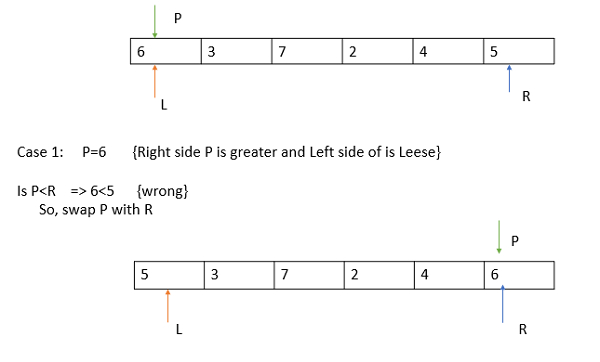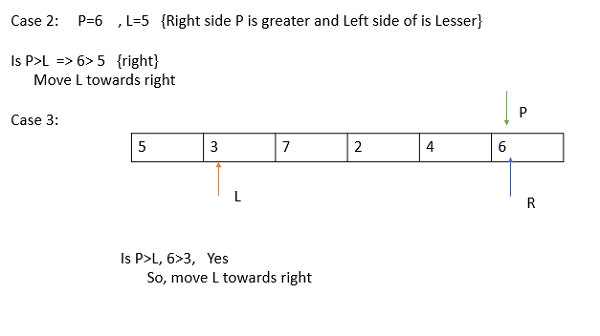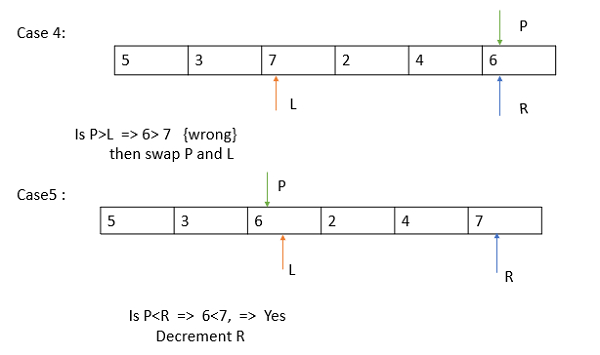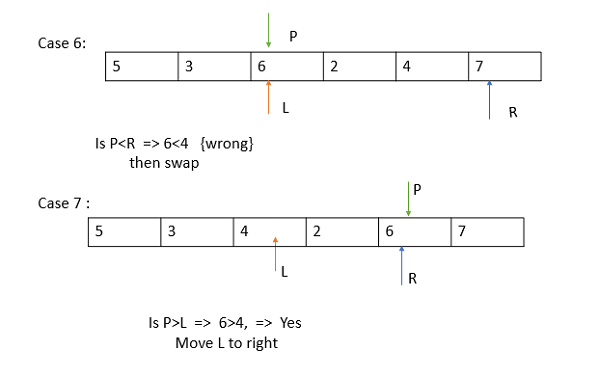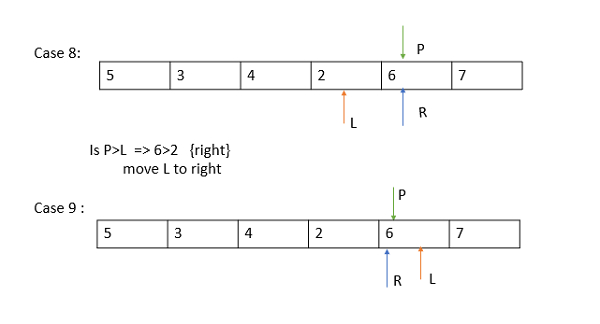Now,

• The pivot is in fixed position.
• All the left elements are less.
• The right elements are greater than pivot.
• Now, divide the array into 2 sub arrays left part and right part.
• Take left partition apply quick sort.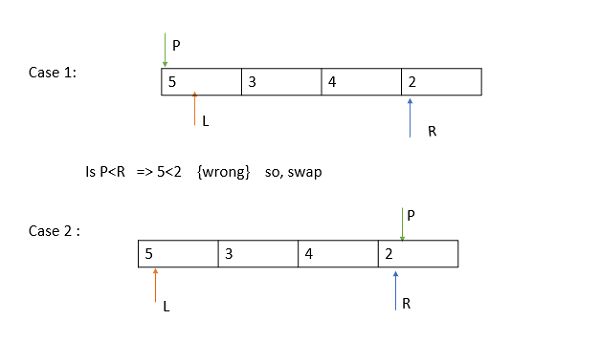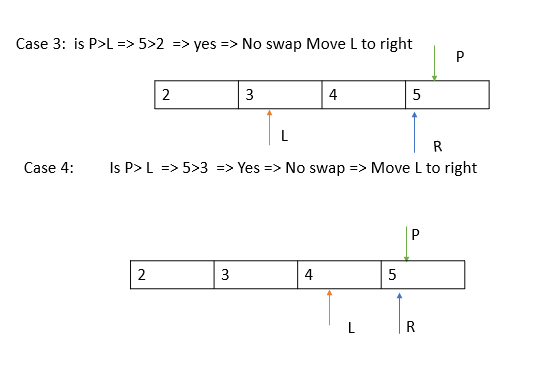Now,

• The pivot is in fixed position.
• All the left elements are less and sorted
• The right elements are greater and are in sorted order.
• The final sorted list is combining two sub arrays is 2, 3, 4, 5, 6, 7

## Example

Following is the C program to sort the elements by using the quick sort technique −

Live Demo

#include<stdio.h>
void quicksort(int number,int first,int last){
int i, j, pivot, temp;
if(first<last){
pivot=first;
i=first;
j=last;
while(i<j){
while(number[i]<=number[pivot]&&i<last)
i++;
while(number[j]>number[pivot])
j--;
if(i<j){
temp=number[i];
number[i]=number[j];
number[j]=temp;
}
}
temp=number[pivot];
number[pivot]=number[j];
number[j]=temp;
quicksort(number,first,j-1);
quicksort(number,j+1,last);
}
}
int main(){
int i, count, number;
printf("How many elements are u going to enter?: ");
scanf("%d",&count);
printf("Enter %d elements: ", count);
for(i=0;i<count;i++)
scanf("%d",&number[i]);
quicksort(number,0,count-1);
printf("Order of Sorted elements: ");
for(i=0;i<count;i++)
printf(" %d",number[i]);
return 0;
}

## Output

When the above program is executed, it produces the following output −

How many elements are u going to enter?: 10
Enter 10 elements: 2 3 5 7 1 9 3 8 0 4
Order of Sorted elements: 0 1 2 3 3 4 5 7 8 9